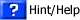Vectors: Reading Questions You can answer these questions on a seperate piece of paper on print off this page. Click here to see the reading assignment. 1. What two “things” make up a vector?2. Below are the two vectors “A” and “B.” Draw the following vectors. Label each one as you draw them, 1/3A, 3A, 1/2B, -2B.3. For the vectors below, draw the resultant when adding A + B. Your answer is a picture of the resultant -no numbers.4. For the vectors below, show the resultant when subtracting A - B. Your answer is a picture of the resultant -no numbers.5. What is the magnitude of each vector shown below?6. What is the direction of each vector shown below? Use the polar coordinate system to describe your answers.by Tony Wayne ...(If you are a teacher, please feel free to use these resources in your teaching.)